# A number 2

A number decreased by the difference between four and the number

Result

y = (Correct answer is: 2x - 4)#### Solution:

$y=x-(4-x) \ \\ y=2x - 4$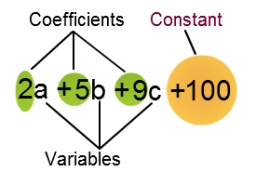We would be pleased if you find an error in the word problem, spelling mistakes, or inaccuracies and send it to us. Thank you!## Next similar math problems:

• Line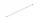How many parts of line divide 5 (different) points that lie on it?
• Change in temperatureStarting temperature is 21°C, the highest temperature is 32°C. What is the change in temperature?
• Temperature change 3At 2 p. M. The temperature was 76 degrees Fahrenheit. At 8 p. M. The temperature was 58 degrees Fahrenheit. What was the change in temperature?
• Math classificationIn 3A class are 27 students. One-third got a B in math and the rest got A. How many students received a B in math?
• Postal stamps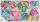Jano and Peter exchanged postal stamps. Jano gave Peter 32 stamps of the missile for 8 stamps with turtles. How good was Jano after this exchange (how many he has surplus in exchanged stamps)?
• SimplifySimplify the following problem and express as a decimal: 5.68-[5-(2.69+5.65-3.89) /0.5]
• Flood waterFlood waters in some US village meant that the homes had to evacuate 364 people. 50 of them stayed at elementary schools, 59 them slept with their friends and others went to relatives. How many people have gone to relatives?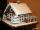Janka and Marienka calculated that there are 210 gingerbreads on the gingerbread house. Janko ate one-seventh of all gingerbreads, and Marienka ate a third less than Janko. How many gingerbreads remained in the gingerbread house?
• The temperature 6The temperature was 47°F on Thursday and 60°F on Friday. How much did the temperature rise?Added together and write as decimal number: LXVII + MLXIV
• SchoolThere are 150 pupils in grade 5 . 2/3 of it are female. By what fractions are the males?
• Minutes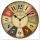Determine the difference in minutes: T1 = 2 3/20 h T2 = 2.3 h
• Roman numerals 2-Subtract up the number written in Roman numerals. Write the results as Roman numbers.
• Bus tickets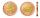Bus ticket for a trip from Prague to Paris cost 2180Kč. A return ticket costs 3930Kč. How much money will save a family of four to go to Paris and back when they purchase a return tickets?
• ExpressionSolve for a specified variable: P=a+4b+3c, for a
• SubtractionTest what do you know about the subtraction of two numbers: a) make a difference if the minuend 4,307,288 and subtrahend is 472008 b) minuend is 4000 more than subtrahend. What's the difference? c) the difference is 38900 less than the minuend. What is th
• EurosPeter spent a quarter of his pocket saving. He has 9 euros now in his wallet . How many euros spent?